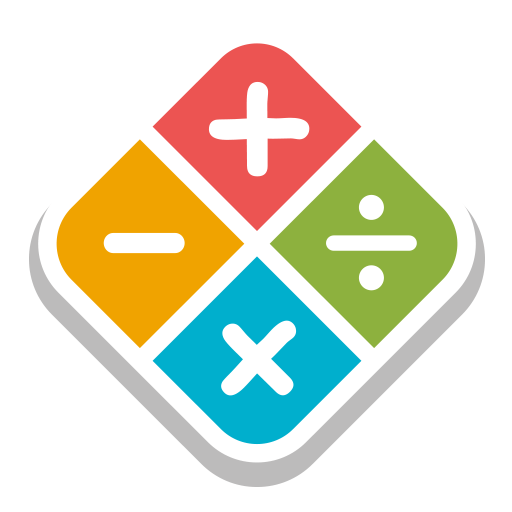# Voting Now

##### Creativity
×## Math Games for Kids

### by kainraziall

Free
Design
Usability
Creativity
Average
7 USERS VOTED
##### TAGS
###### Description

Math Games for Kids

Free math games. Improve your mathematics.

Easy way to learn and practice

1. Addition Table from 1 to 12.
2. Subtraction Table from 1 to 12.
3. Multiplication Table from 1 to 12.
4. Division Table from 1 to 12.

Features :
- Addition games: Adding numbers with learn , practice and test quiz games
- Subtraction games: learn to Subtract numbers and play quiz games
- Multiplication games: Multiplication tables learning and play quiz
- Division games: Practice , learn and test Division tables

With this app you'll just pick a number from 1 to 12 and you'll get its corresponding table so that you can memorize Addition , Subtraction , Multiplication , Division.

It's a cool math game which helps you learn math tables.

math tables will be displayed which makes learning really easy for kids..

Categories: